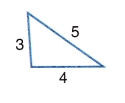# McGraw Hill Math Grade 8 Lesson 20.1 Answer Key Triangles: Acute, Right, Obtuse, Equilateral, Isosceles, and Scalene

Practice the questions of McGraw Hill Math Grade 8 Answer Key PDF Lesson 20.1 Triangles: Acute, Right, Obtuse, Equilateral, Isosceles, and Scalene to secure good marks & knowledge in the exams.

## McGraw-Hill Math Grade 8 Answer Key Lesson 20.1 Triangles: Acute, Right, Obtuse, Equilateral, Isosceles, and Scalene

Exercises

IDENTIFY

Identity as acute, right, or obtuse.

Question 1.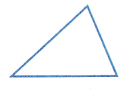Acute Triangle,

Explanation:
An acute angle triangle (or acute-angled triangle) is a triangle in which all the interior angles are acute angles or an acute angle is an angle that is less than 90°.
Given triangle has all interior angles, so it is acute triangle.

Question 2.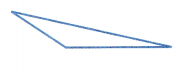Obtuse Triangle,

Explanation:
An obtuse-angled triangle is a triangle in which one of the interior angles measures more than 90° degrees. Given triangle one of interior angle measures
more than 90° degrees, so it is obtuse triangle.

Question 3.Right Triangle,

Explanation:
A right angled triangle is a triangle with one of the angles as 90 degrees.
As given triangle has one angle 90 degress so it is right triangle.

Question 4.Acute Triangle,

Explanation:
An acute angle triangle (or acute-angled triangle) is a triangle in which all the interior angles are acute angles or an acute angle is an angle that is less than 90°.
Given triangle has interior angles less than 90 degress so it is acute triangle.

Question 5.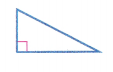Right Triangle,

Explanation:
A right angled triangle is a triangle with one of the angles as 90 degrees.
As given triangle has one angle 90 degress, so it is right triangle.

Question 6.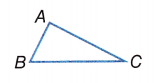Acute Triangle,

Explanation:
An acute angle triangle (or acute-angled triangle) is a triangle in which all the interior angles are acute angles or an acute angle is an angle that is less than 90°.
Given triangle has all interior angles are acute.

Identify as isosceles, scalene, or equilateral.

Question 7.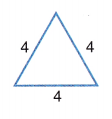Equilateral Triangle,

Explanation:
All the given sides are 4 and equal so given triangle is equilateral triangle.

Question 8.Isosceles Triangle,

Explanation:
As given triangle has 2 sides with 8 and one side with 4 lengths so it is an isosceles triangle. As an isosceles triangle is a triangle that has any two sides equal in length and angles opposite to equal sides are equal in measure.

Question 9.